## Consider a conducting rod of length 34 cm moving along a pair of rails, and a magnetic field pointing perpendicular to the plane of the rail

Question

Consider a conducting rod of length 34 cm moving along a pair of rails, and a magnetic field pointing perpendicular to the plane of the rails.

Required:
At what speed (in m/s) must the sliding rod move to produce an emf of 0.75V in a 1.75T field?

in progress 0
6 months 2021-08-05T17:29:04+00:00 1 Answers 7 views 0

The speed is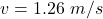Explanation:

From the question we are told that

The  length of the rod is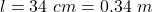The  induced emf is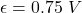The  magnetic field is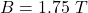Generally the speed is mathematically represented as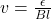=>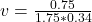=>